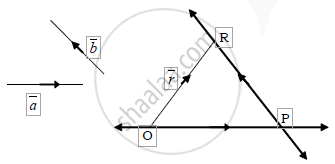# If a and b are any two non-zero and non-collinear vectors then prove that any vector r coplanar with a and b can be uniquely expressed as r=t1a+t2b , where t1 and t2 are scalars - Mathematics and Statistics

If bar a and bar b are any two non-zero and non-collinear vectors then prove that any vector bar r  coplanar with  bar a and bar b can be uniquely expressed as bar r=t_1bara+t_2barb , where  t_1 and t_2  are scalars

#### Solution

Let  bar r = bar(OR) be any vector in a plane.
Let bara and barb be two non-zero non-collinear vectors in the same plane.
We have to prove that barr can be expressed as a linear combination of bara and barb and the linear combination is unique.
Passing through the point O, draw a line parallel to bara, and passing through the point R draw another line || to barb and let them intersect at the point P.therefore bar(OP)|| bara and bar(PR)|| barb

therefore bar(OP)=t_1bara and bar(PR)=t_2 barb

(where  t_1 and t_2 are any two scalars)
In triangle OPR by triangle law

bar(OR)=bar(OP)+bar(PR) " i.e " bar r=t_1bara+t_2barb

Thus barr is expressed as a linear combination of bar a and bar b

To prove the uniqueness of the linear combination.
If possible let  alpha' and beta' be two scalars
Such that:  bar r =alpha' a +beta' b ,

where t_1 ne alpha' and t_2 ne beta'

therefore " we get " t_1bara +t_2 barb=alpha'bara+beta'barb

(t_1alpha')bara=(t_2-beta')barb

Dividing throughout by t_1-alpha'  we get :

bar a=((beta'-t_2)/(t_1-alpha'))barb

This is of the form bar a = k barb , which shows that bar a & bar b are collinear which is a contradiction.
Our assumption that t_1 ne alpha' is wrong.

t_1=alpha' . Similarly, we can prove that t_2=beta'

∴ The linear combination is unique.

Concept: Collinearity and Coplanarity of Vectors
Is there an error in this question or solution?
2012-2013 (March)

Share